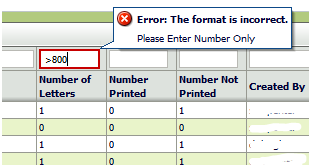Friday, August 29, 2014

Adding a regex QBE validator for numeric columns

I had a table containing a column that was a Number type.  If you tried to filter on a non numeric value a ConverterException would be thrown.   That was fixed by putting a Number converter on the filter input text to allow only numbers.   That worked except now you couldn't use the Query by Example (QBE) Search criteria Operators.These are the supported operators

Operator Description
`>` Greater than
`<` Less than
`>=` Greater than or equal to
`<=` Less than or equal to
`AND` And
`OR` Or

You can write a custom QBE adapter to fix this but that seemed like overkill.

To fix this I changed the filter to be an input text and added a  regular expression validator to allow these operators.  The pattern I used is below.   I didn't find a regex example for QBE Numeric filtering and I'm not a regex expert (is anyone?)  so it took a while to get the regex string just right.  Hopefully this will save someone some time.
Here's the pattern
^[ ]*[<>]?([<=]|[>=])?[ ]*[0-9]+[ ]?(?i)([ ]+AND[ ]+[<>]?[ ]*[0-9]*|[ ]+OR[ ]+[<>]?[ ]*[0-9]*)?[ ]*\$

<f:facet name="filter">

<af:inputText  value="#{vs.filterCriteria.Count}" columns="8" id="Count">

<af:validateRegExp pattern="^[ ]*[<>]?([<=]|[>=])?[ ]*[0-9]+[ ]?(?i)([ ]+AND[ ]+[<>]?[ ]*[0-9]*|[ ]+OR[ ]+[<>]?[ ]*[0-9]*)?[ ]*\$"

</af:inputText>

</f:facet>

<af:outputText value="#{row.Count}"    id="ot7" />
<af:column>

Examples of Things you can put in the filter
10
50
> 10
>10  AND <  50
> 10 and < 50
10 or 50

If the column is a String type (even through it might contain numbers) you don't have this problem and wouldn't need to add a regex Validator.